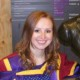# Propagation in the absence of classical spacetimeThe author’s research group busy at work. Madhavan Varadarajan is a Professor at the Raman Research Institute in Bangalore, India.

At the Planck scale of 10−33cm, where the very notion of classical spacetime ceases to exist due to large quantum fluctuations of spacetime geometry, can meaning be given to the notion of “causality”? We are interested in this question in the context of Loop Quantum Gravity (LQG).

The basic quantum states of LQG are labelled by graphs. Each such state describes discrete one dimensional excitations of spatial geometry along the edges of its graph label. These ‘graphical’ states provide the kinematic arena for the quantum theory. The dynamics of LQG is defined by the operator correspondent of the Hamiltonian constraint of classical gravity. Roughly speaking, this Hamiltonian constraint operator acts on kinematic states and does so by changing their underlying graph structure.

Since the notion of causality is a dynamical one, its elucidation is tied up with the properties of the Hamiltonian constraint operator. While the current state of art in LQG does not suffice to provide a complete answer to our question, it is possible to pose and answer an intuitively related question articulated by Smolin : Under the repeated action of the Hamiltonian constraint on a graphical state, do changes in one part of the graph propagate to another part? Smolin noted that repeated operator actions create an independent web of substructure around each vertex of the graph but do not propagate substructures around one vertex of the original graph to any other vertex. This suggests that LQG dynamics may not possess the seeds of a classical description where perturbations such as gravity waves do propagate. The aim of our work is to re-examine Smolin’s question.

Since LQG is a work in progress, we examine this question in a complete quantization of a
simple toy model known as Parameterised Field Theory (PFT). The model is just free scalar field theory on 1+1 flat spacetime, but the classical description is reformulated in a generally covariant disguise by ‘parameterising’ the Minkowskian coordinates and treating them as canonical variables to be varied in the action. Its canonical quantization in an LQG like representation was constructed some time ago. The ensuing quantum structures closely resemble those of LQG but, by virtue of the simplicity of the model, can be interpreted and manipulated with ease. The kinematic graphical states of the representation admit an interpretation as quantum excitations of the scalar field on discrete Cauchy slices in a lightcone latticization of the classical flat spacetime. As in LQG, the dynamics is defined by a Hamiltonian constraint operator, whose repeated action does not propagate graph sub structures from one vertex to another. In the language of discrete Cauchy slices, it can be seen that there is no propagation beyond one lattice time step.

Nevertheless, there is a precise sense in which graph substructures do propagate and it has to do with the physical states of the theory. Physical states are those linear combinations of kinematical ones which satisfy an appropriate notion of ‘quantum general covariance’. Such a ‘generally covariant’ physical state must lie in the kernel of the Hamiltonian constraint because the action of the Hamiltonian constraint is intimately connected with the ‘time reparameterization’ aspect of general covariance. It turns out that each such generally covariant quantum state is an infinite sum of ‘discrete Cauchy slice’ kinematic states. There are summands in this sum whose graph labels are related by the propagation of graph structures and which represent discrete slices seperated by any number of lattice time steps between which quantum matter excitations do propagate. Indeed, the Cauchy slices in this sum all stack up into a single discrete spacetime lattice and the quantum matter excitations on these slices propagate on this discrete spacetime. Thus, propagation is seen as a property of physical states rather than that of repeated actions of the Hamiltonian constraint on kinematic states.

In our work we unearth the structural properties of the PFT Hamiltonian constraint responsible for physical ‘discrete spacetime’ states being in its kernel despite it not generating propagation through its repeated action on kinematic ‘discrete’ space states. We would like to import these structural lessons to the context of LQG and reframe Smolin’s question of propagation as follows: does the putative sum over kinematic states which is to represent a generally covariant physical state contain kinematic states which are related by the propagation of graph substructures? The answer to this question depends on the definition of the Hamiltonian constraint because, as mentioned above, generally covariant states must lie in the kernel of this operator. The definition of the Hamiltonian constraint operator is a key open issue in LQG so that a search for an affirmative answer is intimately related to progress on this open issue.

 L. Smolin, e-Print: gr-qc/9609034
 A. Laddha and M. Varadarajan, Phys. Rev. D 83 025019 (2011)

Propagation in Polymer Parameterised Field Theory